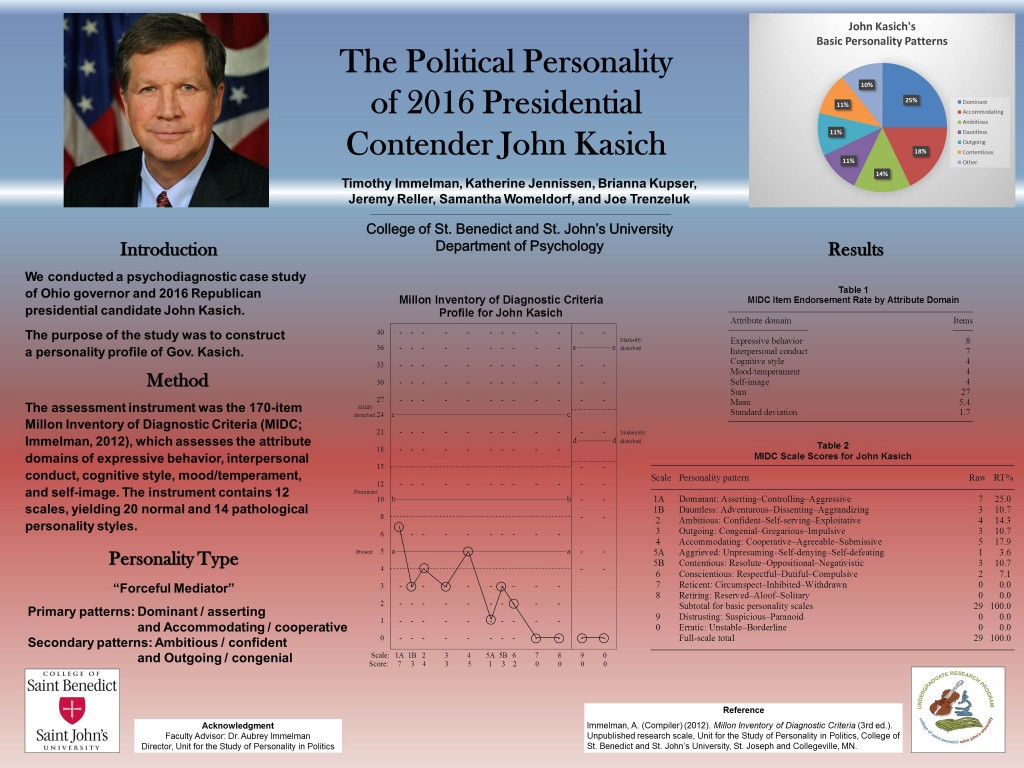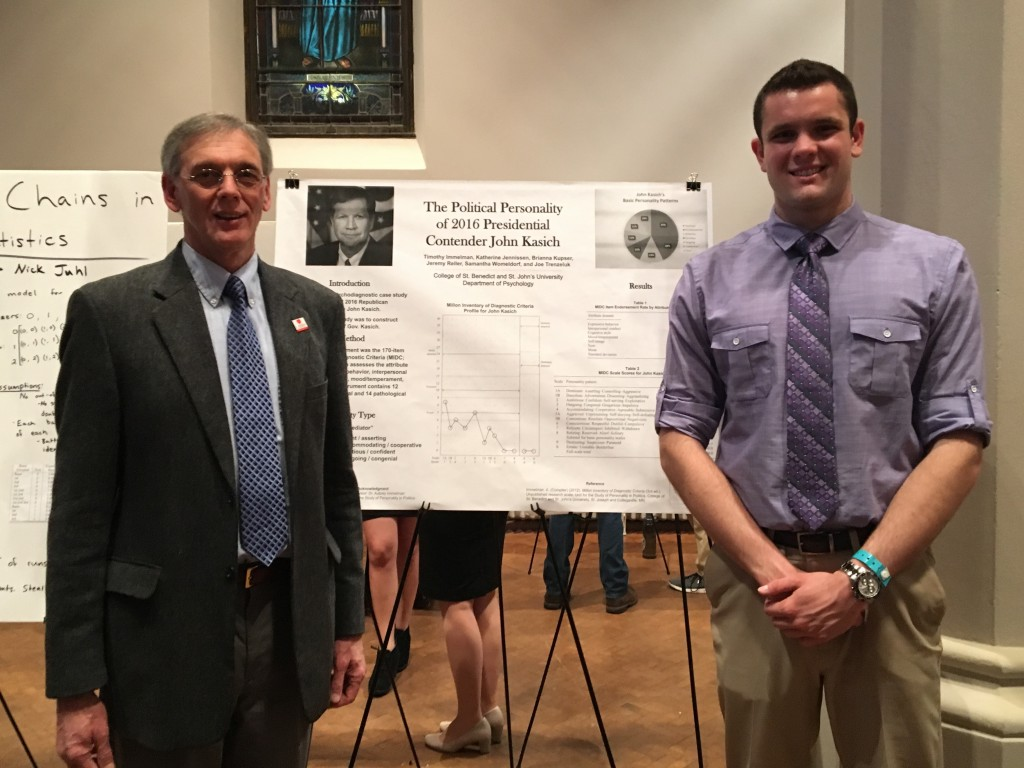# John Kasich

## The Personality Profile of 2016 Republican Presidential Candidate John Kasich

April 18, 2016

A psychological analysis of Ohio governor John Kasich — a contender for the Republican nomination in the 2016 presidential election — by Timothy Immelman, Katherine Jennissen, Brianna Kupser, Jeremy Reller, Samantha Womeldorf, Joe Trenzeluk, and Aubrey Immelman, Ph.D., at the Unit for the Study of Personality in Politics, revealed that Kasich’s primary personality patterns are Dominant/asserting and Accommodating/cooperative, with secondary Ambitious/confident and Outgoing/congenial features. In summary, Kasich’s personality composite can be characterized as a forceful mediator. (Note: This is a pilot study with a lower confidence level than comparable studies of Donald Trump, Ted Cruz, and Hillary Clinton.)Click on image for larger view

#### Note: Personal Electability Index (political impact) score

John Kasich scores relatively low on the Millon Inventory of Diagnostic Criteria-based Personal Electability Index, which has accurately predicted the outcome of every presidential election since 1996.

Following are the PEI calculations for John Kasich:

John Kasich: PEI = 14

 Scale: 1A 1B 2 3 4 5A 5B 6 7 8 Score: 7 3 4 3 5 1 3 2 0 0

Scale: 1A = 7; 2 = 4; 3 = 3; 6 = 2; 8 = 0

[Extraversion (scale 3) = 3] + [Narcissism (scale 2) = 4] + [Dominance (scale 1A) = 7] – [Introversion (scale 8) = 0] – [Conscientiousness (scale 6) = (2 – 2) = 0] = 14 – 0 = 14

Following are the PEI calculations for Kasich’s rivals:

Bernie Sanders: PEI = 18

 Scale: 1A 1B 2 3 4 5A 5B 6 7 8 Score: 13 5 6 3 5 0 8 3 0 4

Scale: 1A = 13; 2 = 6; 3 = 3; 6 = 3; 8 = 4

[Extraversion (scale 3) = 3] + [Narcissism (scale 2) = 6] + [Dominance (scale 1A) = 13] – [Introversion (scale 8) = 4] – [Conscientiousness (scale 6) = (4 – 4) = 0] = 22 – 4 = 18

Ted Cruz: PEI = 25

 Scale: 1A 1B 2 3 4 5A 5B 6 7 8 Score: 13 6 8 4 0 0 4 2 0 0

Scale: 1A = 13; 2 = 8; 3 = 4; 6 = 2; 8 = 0

[Extraversion (scale 3) = 4] + [Narcissism (scale 2) = 8] + [Dominance (scale 1A) = 13] – [Introversion (scale 8) = 0] – [Conscientiousness (scale 6) = (2 – 2) = 0] = 25 – 0 = 25

Hillary Clinton: PEI = 29 (dysfunctionality adjusted)

 Scale: 1A 1B 2 3 4 5A 5B 6 7 8 Score: 15 2 15 1 0 0 0 6 0 0

[Extraversion (scale 3) = 1] + [Narcissism (scale 2) = 15] + [Dominance (scale 1A) = 15] – [Introversion (scale 8) = 0] – [Conscientiousness (scale 6) = (6 – 4)  = 2] = 31 – 2 = 29

Donald Trump: PEI = 45 (dysfunctionality adjusted)

 Scale: 1A 1B 2 3 4 5A 5B 6 7 8 Score: 19 11 24 19 1 0 6 2 1 0

Scale: 1A = 19; 2 = 24; 3 =19; 6 = 2; 8 = 0

[Extraversion (scale 3) = 15] + [Narcissism (scale 2) = 15] + [Dominance (scale 1A) = 15] – [Introversion (scale 8) = 0] – [Conscientiousness (scale 6) = (2 – 2) = 0] = 45 – 0 = 45

### UpdateResearch director Aubrey Immelman (left) with Tim Immelman (right), who presented “The Political Personality of 2016 Presidential Contender John Kasich” (by Timothy Immelman, Katherine Jennissen, Brianna Kupser, Jeremy Reller, Samantha Womeldorf, and Joe Trenzeluk) at Scholarship and Creativity Day, College of St. Benedict and St. John’s University, April 21, 2016. (Click photo for full-size image)